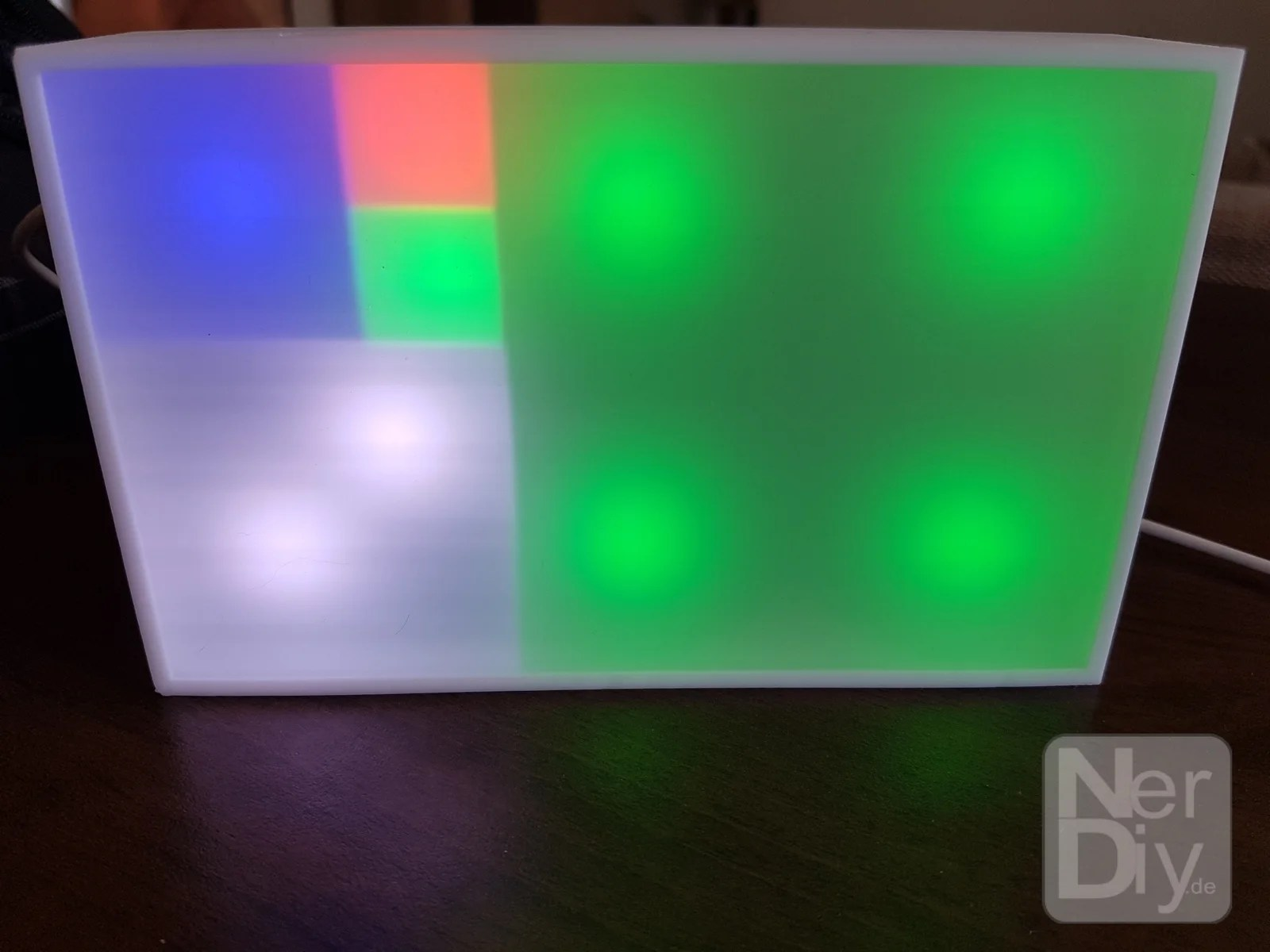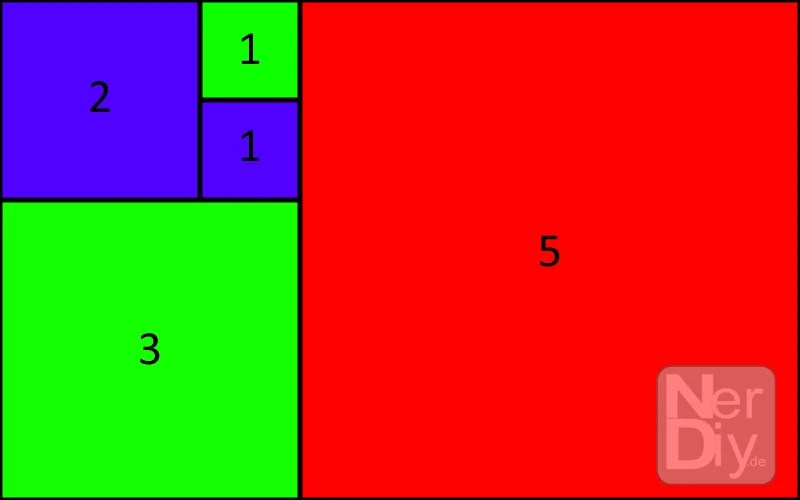# FibonacciClock – An unusual time indicator

A few months ago I came across a project by Philippe Chrétien (geekoclock.com) in 2015 at Kickstarter. There (www.kickstarter.com/projects/basbrun/fibonacci-clock-an-open-source-clock-for-nerds-wit) a watch was offered for sale showing the time using the Fibonacci series.

For those who do not know what the Fibonacci episode is here is a little digression:
In the Fibonacci sequence, the next number is always the sum of the two previous (Fibonacci) numbers. So if you start at 1 it is 1, 1, 2, 3, 5, …

And with these five numbers that the Fibonacci clock can represent the current time.
The dial consists of LED surfaces that glow in different colors and thus represent the respective numerical values from which the current time can be read off.

As always, this can be explained best by a few examples.

Hints for our lovely english readers: Basically, many of the articles on Nerdiy.de are translations from the original german articles. Therefore, it may happen here and there that some illustrations are not available in english and that some translations are weird/strange/full of mistakes or generally totaly wrong. So if you find some obvious (or also not obvious) mistakes don't hesitate to leave us a hint about that in the comment section.
Also please don't get confused, that instead of a "dot" often a "comma" is used as decimal separator. 🙂

## Display der FibonacciUhrThe “display” of the Fibonacci clock is divided into five areas of which each area can glow in a different color.

In order to read the time of the Fibonacci clock a few basics needs to be explained first. On this picture you can see the distribution of the display of the FibonacciClock. This is divided into five areas. Each of these surfaces has the specified significance. The large red surface marked with a “5” has the valency five, the next smaller green glowing surface the valence three, the next smaller blue luminous surface the valence two and the other two surfaces each the valence one.

If you pay close attention, you will surely notice that the numbers 1 to 12 can be displayed which – surprise – is perfect for displaying the number of hours in a clock.
If, for example, only the area with the value five lights up, it is just five o’clock. If, in addition to the area with the value five, the area with the value two also lights up, these must be added together. This would then correspond to the time seven (2 + 5) o’clock.

In this way, all times can be from 0 (it does not illuminate any area) to 12 (all surfaces are illuminate).

All right, now we know how to read and “calculated” the hours, but how do you know now the number of minutes of the displayed time?
Now the different colors come into play. As you may have noticed, the areas glow in either blue, red or green.
And this helps you tu also show the minutes. For example, the clock indicates whether the current value is just for the number of hours, just the number of minutes, or both.

If an area is red, its value may only be taken into account for the number of hours.
If a surface glows green, its value may only be taken into account for the number of minutes.
On the other hand, if an area is blue, its value must be taken into account for both, the number of hours and the number of minutes.

But we are not done yet, the number of minutes read must now be multiplied by five.
And there we are already at the first small disadvantage of the clock.
Apart from the fact that one needs – just at the beginning – a bit longer until you have read the time, the minutes can only be displayed in five-minute increments. That means for example, that the clock shows the real time of 08:43 as 08:40. The display thus always rounds off to the previous value divisible by five.

## Examples

That sounds first pretty complicated now. Once understood, however, you quickly realize that it is not.
For a better understanding we will try to read the displayed time of the example.In the shown display, the areas will light up as follows:
Area with significance 5: Red = valence applies only to the number of hours
Area of significance 3: Green = valence applies only to the number of minutes
Area with significance 2: Blue = valence applies to the number of hours and the number of minutes
Area with significance 1: Green = valence applies only to the number of minutes

Now you just have to summarize the valences:
Hours: 5 + 2 = 7
Minutes: 3 + 2 + 1 = 6 and this multiplied by five gives: 30

The displayed time is therefore 07:30.

I think this is a great project for anyone who is interested in numbers and puzzles, and I also find a great gift idea for engineers, mathematicians, teachers and scientists in general.

To practice here are a few displays with the respective time displayed

This slideshow requires JavaScript.

## Build NerdiysFibonacciClock yourself

After all the theory about this clock, it was clear to me that I also had to have one. However, I did not just want to rebuild the hardware of the clock and instead have my own design considered which can be easily copied.

For all those who also want to build their own Fibonacci watch, I have logged the structure and the programming. You can also find a simple user manual at the following links. 🙂

You can get the required parts in the shop under https://www.nerdiy.de/produkt-kategorie/fibonacciuhr/.